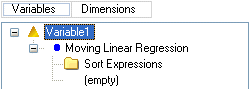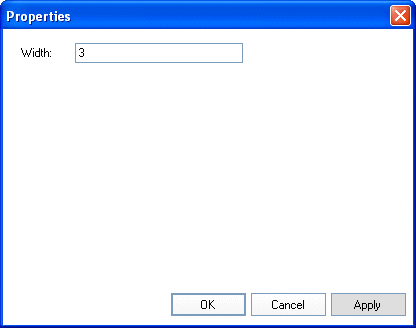# Moving Linear Regression - Teradata Warehouse Miner

Product
Release Number
5.4.6
Published
November 2018
Language
English (United States)
Last Update
2018-12-07
dita:mapPath
gxn1538171534877.ditamap
dita:ditavalPath
ft:empty
dita:id
B035-2301
Product Category
Software

The non-standard Teradata specific moving linear regression function is supported, generating MLINREG (expression, width, sort expression). The function will work without any special enhancement when applied to a date expression. MLINREG may not be mixed in the same analysis with aggregation functions such as average, and partitioning is not supported.

Given a single expression, width, and sort expression, this Ordered Analytical function derives a new column giving the moving linear regression extrapolation of the expression over “width” rows when sorted by the sort expression, using the sort expression as the independent variable. The current and “width-1” rows after sorting are used to calculate the simple least squares linear regression. For rows that have less than “width-1” rows preceding it in the table or group, the function is computed using all preceding rows. The first two rows in the table or group, however, will have the NULL value.

As an example, moving linear regression predicting y based on x over w rows looks like the following.

`MLINREG(y, w, x)`

When dragging a Moving Linear Regression function into a variable, the following tree element is created.

Variable Creation > Input > Variables: SQL Elements pane - Logical > Moving Linear RegressionA single sort expression should be placed in the Sort Expressions folder, and a column or expression should be moved into the (empty) branch of the tree. The Width is specified using the Properties panel. Double-click on Moving Linear Regression, or highlight it and click Properties.

Variable Creation > Input > Variables: SQL Elements pane - Logical > Moving Linear Regression PropertiesThe default Width of 3 can be updated here.